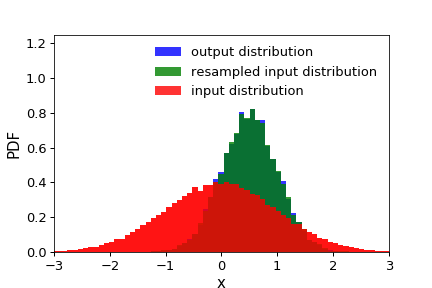# distribution_matching_indices¶

halotools.utils.distribution_matching_indices(input_distribution, output_distribution, nselect, bins, seed=None)[source] [edit on github]

Calcuate a set of indices that will resample (with replacement) input_distribution so that it matches output_distribution.

This function is useful, for example, for comparing a pair of samples with matching stellar mass functions.

Parameters: input_distribution : ndarray Numpy array of shape (npts1, ) storing the distribution that requires modification output_distribution : ndarray Numpy array of shape (npts2, ) defining the desired output distribution nselect : int Number of points to select from input_distribution. bins : ndarray Binning used to estimate the PDFs. Default is 100 bins automatically determined by numpy.histogram. seed : int, optional Random number seed used to generate indices. Default is None for stochastic results. indices : ndarray Numpy array of shape (nselect, ) storing indices ranging from [0, npts1) such that input_distribution[indices] will have a PDF that matches the PDF of output_distribution.

Notes

Pay careful attention that your bins are appropriate for your two distributions. The PDF of the returned result will only match the output_distribution PDF tabulated in the input bins. Depending on the two distributions and your choice of bins, may not be possible to construct matching PDFs if your sampling is too sparse or your bins are inappropriate.

Examples

>>> npts1, npts2 = int(1e5), int(1e4)
>>> input_distribution = np.random.normal(loc=0, scale=1, size=npts1)
>>> output_distribution = np.random.normal(loc=.5, scale=0.5, size=npts2)
>>> nselect = int(2e4)
>>> bins = np.linspace(-2, 2, 50)
>>> indices = distribution_matching_indices(input_distribution, output_distribution, nselect, bins)